Pandas read_csv() function provides a straightforward way to read data from a CSV file and convert it into a DataFrame.

## Syntax

``````pd.read_csv(filepath_or_buffer, sep=’ ,’ , header=’infer’,
index_col=None, usecols=None, engine=None,
skiprows=None, nrows=None)
``````

## Parameters

1. sep or delimiter: Specifies the delimiter used to separate fields in the CSV file (default is ,).
2. header: Indicates the row number(s) to use as the column names (default is 0, meaning the first row is used as column names). If there are no column names in the file, set header=None.
3. index_col: Specifies the column(s) to use as the index (row labels) of the DataFrame.
4. names: Specifies a list of column names to use when header=None.
5. skiprows: Skips a specified number of rows or a list of row indices to skip while reading the file.
6. na_values: Specifies additional strings to recognize as NaN (Not a Number) or missing values.
7. dtype: Specifies the data type for one or more columns, provided as a dictionary.

For this example, we will use the mlb_players.csv file.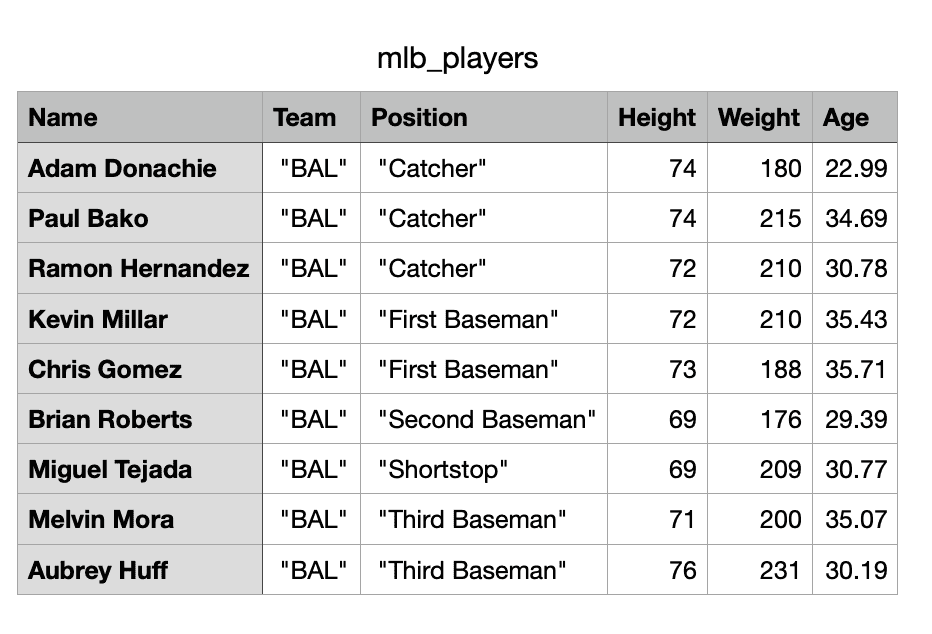To import this csv file and convert it into a DataFrame, you must use the “pandas.read_csv()” function.

``````# Import pandas
import pandas as pd

print(df)
``````

Output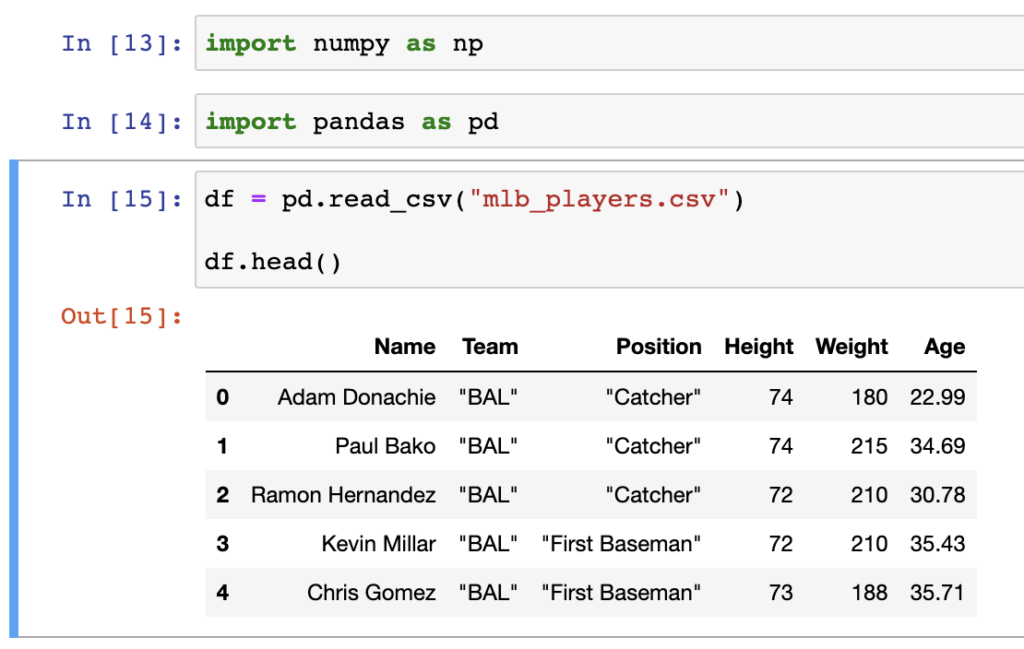## Example 2: Using ‘usecols’ in read_csv()

In this code, we are specifying only 3 columns,i.e.[‘Name’, ‘Position’, ‘Age’], to load, and we use the header 0 as its default header.

``````# Import pandas
import pandas as pd

usecols=['Name', 'Position', 'Age']
)

Output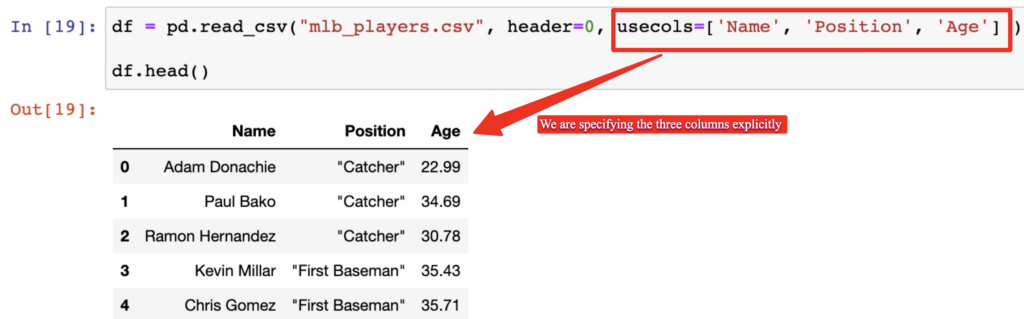## Example 3: Using index_col in read_csv()

``````# Import pandas
import pandas as pd

usecols=['Name', 'Position', 'Age'],
index_col=['Name', 'Age'],
)

Output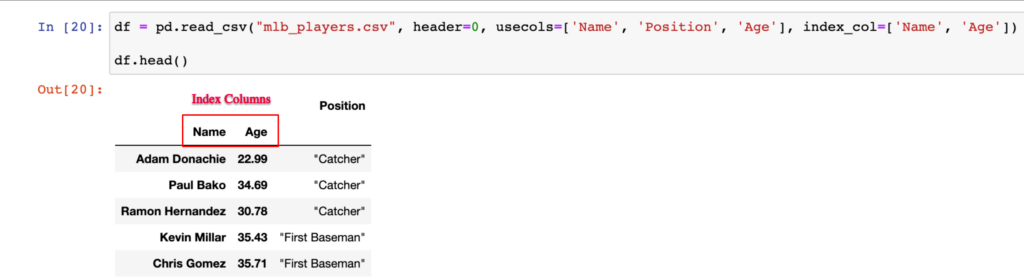## Example 4: Using nrows in read_csv()

We can just display only 4 rows using the nrows parameter.

``````# Import pandas
import pandas as pd

usecols=['Name', 'Position', 'Age'],
index_col=['Name', 'Age'],
nrows=4
)

df``````

Output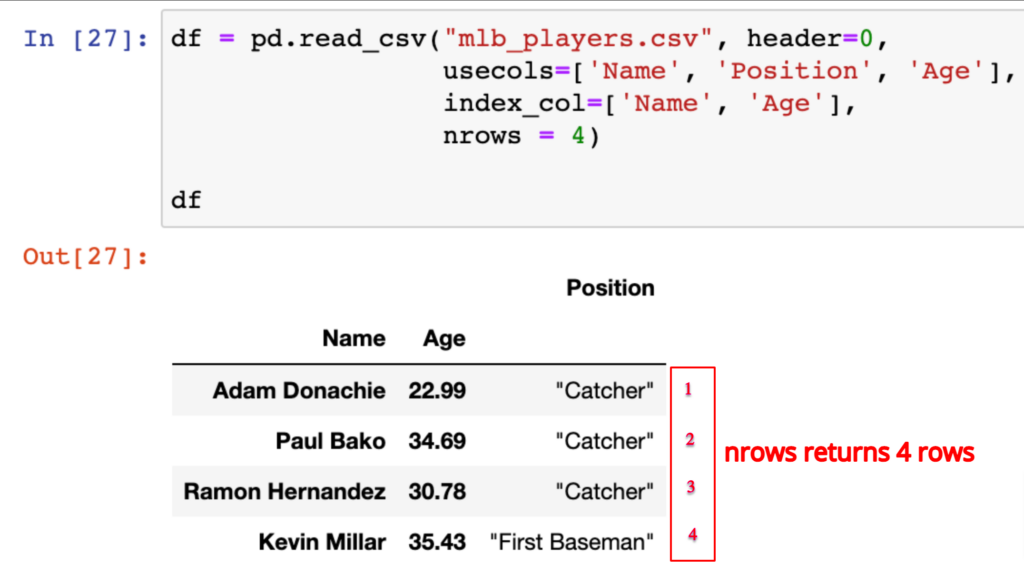## Example 5: Using skiprows in read_csv()

### Before skipping the rows

``````# Import pandas
import pandas as pd

df``````

### After skipping the rows

``````# Import pandas
import pandas as pd

skiprows=[1, 3]
)

df``````

Output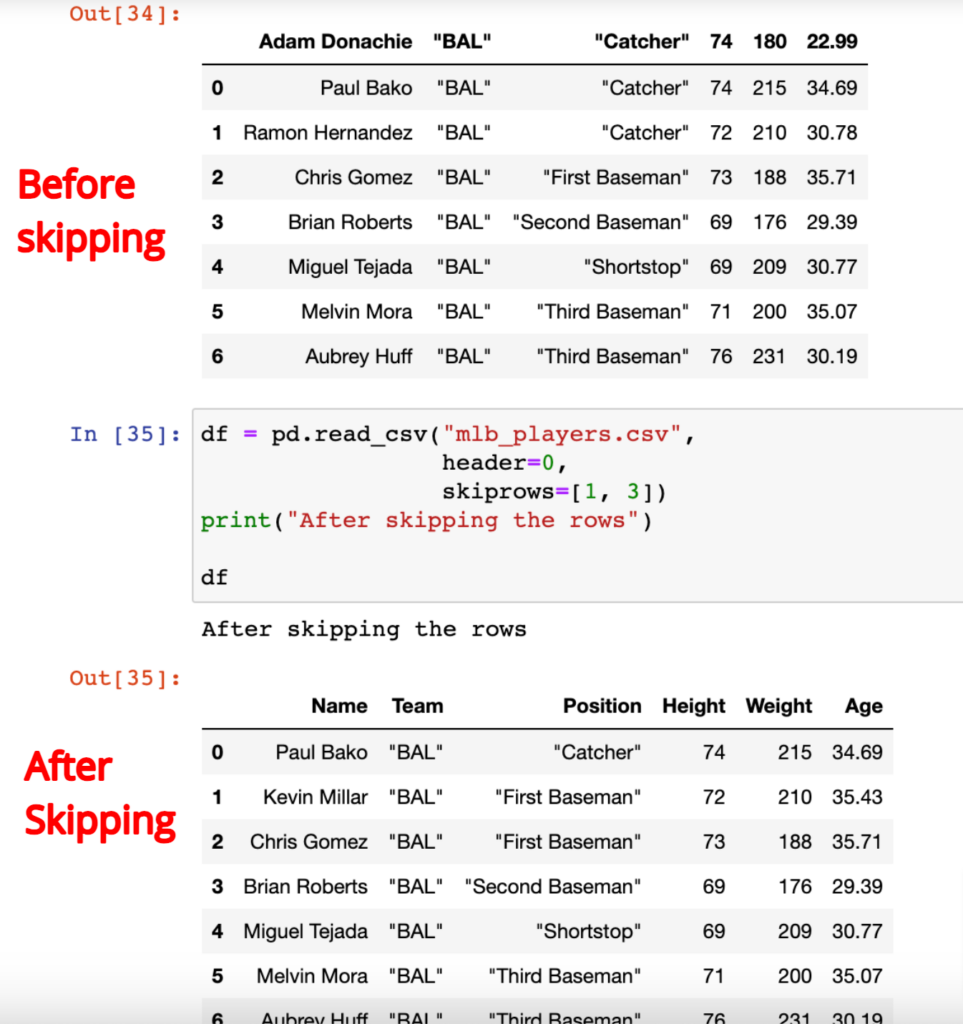That’s it.

### 1 thought on “Pandas.read_csv() Method”

1.python3 issue with NaN … df shows NaN but df1 shows .
Since I pass na_values=[‘.’], I expect df to show me .